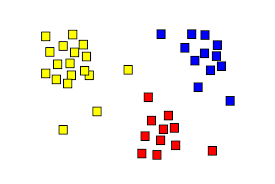# 3 minute read to ‘How to find optimal number of clusters using K-means Algorithm’

Original article was published by Kavya Gajjar on Becoming Human: Artificial Intelligence MagazineUsually in any K-means clustering problem, the first problem that we face is to decide the number of clusters(or classes) based on the data. This problem can be resolved by 3 different metrics(or methods) that we use to decide the optimal ‘k’ cluster values. They are:

1. Elbow Curve Method
2. Silhouette Score
3. Davies Bouldin Index

Let us take a sample dataset and implement the above mentioned methods to understand their working.

We will use the make blobs dataset from sklearn.datasets library for illustrating the above methods

Now let’s look at what these methods area and that after implementing those three methods on the created dataset what are the results.

A) Elbow Curve
The main idea is to define clusters such that the total within-cluster sum of square (WSS) is minimized. It measures the compactness of the clustering and we want it to be as small as possible. The idea is to choose a number of clusters (k) so that adding another cluster doesn’t improve much better the total WSS.

***What Within-cluster Sum of Square mean (WSS)?
Basically it is the sum of squared distance (usually Euclidean distance) from it’s nearest centroid (center point of cluster).

It decreases with increasing number of clusters(k). Aim is to find the bend (like an elbow joint) point in the graph.

### Trending AI Articles:

1. Fundamentals of AI, ML and Deep Learning for Product Managers

2. The Unfortunate Power of Deep Learning

3. Graph Neural Network for 3D Object Detection in a Point Cloud

4. Know the biggest Notable difference between AI vs. Machine Learning

***This method is called elbow curve because the visual representation of the WSS w.r.t. the number of clusters(k) looks like human elbow

Here, we find that the k=3 is the bend(elbow) point.
*** Usually elbow curve method is a little ambiguous as the bend point for some datasets is not visible clearly

B) Silhouette Score
Silhouette Score is calculated using mean of intra-cluster distance (a) and the mean of nearest-cluster distance (b) for each sample. The Silhouette Coefficient for a sample is (b -a) / max(a, b). For better clarification, intra-cluster distance (a) is distance of sample point to it’s centroid and (b) is distance of sample point to nearest cluster that it is not a part of. Hence, we want the silhouette score to be maximum. Thus, have to find a global maxima for this method.

Silhouette coefficient exhibits a peak characteristic as compared to the gentle bend in the elbow method. This is easier to visualize and reason with.

Here, we can easily visualize the peak point at k=3.

C) Davies Bouldin Index
It is defined as a ratio between the cluster scatter and the cluster’s separation. Basically a ratio of within-cluster distance and between cluster distances. Aim is to find optimal value in which clusters are less dispersed internally and are farther apart fro each other (i.e. distance between two clusters is high). Hence, a lower value of Davies Bouldin index will mean that the clustering is better.

As I mentioned earlier lower value is desired, so we find the global minima point i.e. k= 3.

So after using all the above mentioned methods, we concluded that optimal value of ‘k’ is 3. Now, implementing the k-means clustering algorithm on the dataset we get the following clusters.

We can see from the above graph that all points are classified into three clusters appropriately. Hence, the k=3 was an optimal value for clustering.

You can find the entire code snippet at GitHub location here: https://github.com/kavyagajjar/K-Means-Clustering/blob/main/K-means-Make_blobs/Optimal_K_using_k_means_clustering.ipynb

### Don’t forget to give us your 👏 !3 minute read to ‘How to find optimal number of clusters using K-means Algorithm’ was originally published in Becoming Human: Artificial Intelligence Magazine on Medium, where people are continuing the conversation by highlighting and responding to this story.